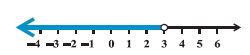Email us to get an instant 20% discount on highly effective K-12 Math & English kwizNET Programs!

#### Online Quiz (WorksheetABCD)

Questions Per Quiz = 2 4 6 8 10

### High School Mathematics - 27.11 Graphical Representation of Solutions of Inequalities

 Example 1: Solve 7x+3 < 5x+9 Solution: We have 7x+3 < 5x+9 2x < 6 or x < 3Example 2: The marks obtained by a student in his exams are 62 and 48. What marks should he get in his next test to get an average of 60? Solution: (62+8+x)/3 > = 60 x > = 70 Answer: The student must obtain a minimum of 70 marks to get an average of 60.
 Q 1: IQ of a person is given by the formula IQ = MA/CA * 100 where MA is mental age and CA is chronical age. If 80 <= IQ <= 140 for a group of 12 year children, find the range of their mental age.More than 16.8Atleast 9.6 but not more than 16.8Less than 9.6 Q 2: The marks obtained by a student in two tests were 70 and 75, what should he score in his third test to get an average of 60?354045 Question 3: This question is available to subscribers only! Question 4: This question is available to subscribers only!